IF YOU CAN SEE THIS go to /sfa/Nuprl/Shared/Xindentation_hack_doc.html

### Pairs - <a,b>

Nuprl's standard pair forming operation is <a,b>. The basic destructor for pairs, sometimes called spread, supplies a pair's components to a function, and is expressed as an operator binding two variables

p/x,yf(x;y)

which evaluates thus:

<a,b>/x,yf(x;y) *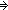f(a;b)

The projection functions are special cases:

p.1 is p/x,yx
p.2 is p/x,yy

<a,b>.1 *a
<a,b>.2 *b

The "cartesian product" type A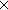B comprises pairs <a,b> where a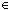A and bB. This is really just a special case of u:AB(u) in which variable u does not occur free in the second type expression. The type u:AB(u) comprisese pairs <a,b> where aA and bB(a). The expression u:AB(u) denotes a type when A denotes a type and, further, B(u) denotes a type-valued function in u:A. Thus, if A denotes the empty type, then u:AB(u) does too, no matter what B(u) is (so long as no variables other than u are free in B(u)).
This type constructor is often called the "general sum" of a family of types, and indeed the most common notation for it is "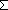u:AB(u)".

Here's a theorem about pairing projections, complementing the above computational facts about projecting off of pairs:

Thm*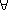B:(A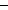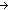Type), p:(u:AB(u)). p = <p.1,p.2>a:AB(a)

Tupling.

We adopt the convention for forming standard tuples by iterating through the second component, so the triple <a,b,c> is a pair having <b,c> as its second component. The notation for spreading tuples is extended as well.a:Ab:Bc:C. <a,b,cABC

or more generally,A:Type, B:(AType), C:(x:AB(x)Type), a:Ab:B(a), c:C(a,b).
<a,b,cu:Av:B(u)C(u,v)

The notation for spreading tuples is extended as well:

t/x,y,zf(x,y,z) abbreviates t/x,##/y,zf(x,y,z)

hence

<a,b,c>/x,y,zf(x,y,z) *<b,c>/y,zf(a,y,z) *f(a,b,c).

(Aug 2003 - sfa )